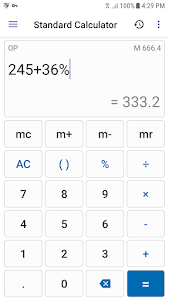# NT Calculator - Extensive Calculator Pro v3.8 (Paid)

### NT Calculator - Extensive Calculator Pro / Screenshots### NT Calculator - Extensive Calculator Pro / Description

Dear you

This Extensive Calculator app allows you to easily handle all the calculations necessary for everyday life with a single application. A Calculator app with a simple interface and practical functions! It can replace your default calculator.

This calculator app don't require ANY PERMISSION on your phone. And it is light and fast.

With Extensive Calculator Pro on your Android you do not need to bring your calculator anymore. This calculator app serves you everything from A - Z.

List of calculators currently supported:
1. Standard Calculator
• Quick and easy.
• Basic features such as: % + - x / 0 - 9 ( and ).
• Memory function such as: M+ M- MR MC.

2. Scientific Calculator
• All features such as: sin cos tan % ! 0-9 ( ) RAD and DEG log ln Mod + - x / SQRT SQR signed...
• You can use EXP is E (sam as 10 ^ ) to calculate large decimal number. If you need to get previous result of calculator you can use ANS button.

• Covert from one thing to the other things is very simple.
• Base 2 8 10 16

4. Time Calculator
• Three primary function is hour (h) minute (m) second (s) millisecond (s) with basic features such as: % + - x / 0 - 9 ( and ).
• History available. And you can reuse by pressing calculation history (this feature is available in standard scientific and time calculator).

5. Loan Calculator
• Suitable for car bank loan
• Three primary input are loan amount interest rate loan term.

6. Date Calculator
• Calculate difference between of two date.

7. Unit Converter
Supports many Unit of measurement commonly used in everyday life such as:
• Weight converter: kg to st lbs to grams lbs to kilograms ...
• Length converter: size converter meters to kilometers ...
• Speed converter: km/h cm/s m/s m/s to km/h ...
• Temperature converter: c to f f to c c to kelvin ...
• Data converter: byte to bit bit to byte ...
• Area converter: km2 to m2 1 hectare to km2 cm2 to m2 ...
• Time converter: minute to second year to second ...
• Energy converter: joule kwh ...
• Pressure converter: atmospheres bars kilopascals pascals ...
• Volume converter: gallons to qts teaspoon cups liters cubic feet ...
• Fuel Converter: many units...

8. Support function in this calculator
• Format number type: 1234.56 or 1.23456.
• Percentage method calculation:
- 100 + 5% = 105 and 100 x 5% = 500.
- 100 + 5% = 100.5 and 100 x 5% = 5.
- 100 + 5% = 100.05 and 100 x 5% = 5.
• Rounding number: from 0 to 10.
• Operation priority:
- 2 + 2 x 2 = 6 (OP).
- 2 + 2 x 2 = 8 (WOP).

Thank you for using the Calculator app if you have questions I am always ready to answer you. I think you will find this app useful and use it with pleasure.

The list will be increased!

### NT Calculator - Extensive Calculator Pro / What's New in v3.8 (Paid)

- ★ Untouched Paid apk with Original Signature
➡ Languages: Multi Languages
➡ CPU architectures: Universal
➡ Screen DPIs: 120dpi, 160dpi, 240dpi, 320dpi, 480dpi, 640dpi

@derrin

Thank you for choosing this app <3 What's new:
1. Add vibration option in app settings.